## -BRAINLIEST IF ANSWERED RIGHT- Given the equation 5+x−12=x−7: Part A. Solve the equation 5+x−12=x−7. I

Question

Given the equation
5+x−12=x−7:

Part A. Solve the equation
5+x−12=x−7. In your final answer, be sure to state the solution and include all of your work.
Part B. Use the values
x=−4,0,5 to verify your solution to the equation
5+x−12=x−7.

in progress 0
5 months 2021-08-15T00:08:02+00:00 1 Answers 10 views 0

Part A: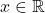(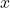is equal to all real numbers)

Part B: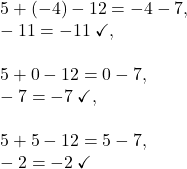Step-by-step explanation:

Part A:

Given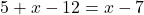, combine like terms: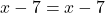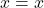Since this is merely a true statement for all real numbers (reflexive property), this equation is true for any real value of.

Therefore,(is equal to all real numbers).

Part B:

Using arbitrary values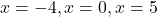as requested in part B, verify: